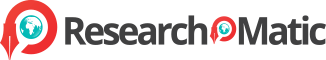﻿ Free academic library on Mathematics & More|Researchomatic
Javascript is disabled. Please enable it for better working experience.

#4,896,000Research-based Topics
2,943,921 VALUED MEMBERS

# Mathematics

Mathematics is the branch of science dealing with the study of numbers and quantities and their interrelationship using symbols and notations. Researchomatic offers you wide range of sample essays on mathematics and other related topics like calculus, algebra, geometry, trigonometry, etc.

## Researchomatic

has the World's largest database of Mathematics topics that help Students, Researchers & Teachers develop multiple academic product

# Top 1 Mathematics Topic

Mathematics

MATHEMATICS

Mathematics

Mathematics

Solution for Problem No. 56

a

=

100

50

2

From cross multiplication, we can find the extreme-mean;

2

a

=

50

*

100

2

a

=

5000

a

=

5000

2

a

=

2500

Thus, the proportion of bear is 2500.

Solution for Problem No. 10

y

-

1

=

-

3

x

+

3

4

Multiply each side by x + 3, in order to find the value of y for the given equation and to bring extraneous variable that is x on the right hand side;

(x + 3)

.

y

-

1

=

(x + 3)

.

-

3

x

+

3

4

From simplifying the above equation, we find;

y

-

1

=

-

3

x

-

9

4

4

Now, add 1 on each side of the equation, we find;

y

-

1

+

1

=

-

3

x

-

9

+

1

4

4

y

=

-

3

x

-

9

+

1

4

4

y

=

-

3

x

-

9

+

4

4

y

=

-

3

x

-

5

4

References

Brandenberger, B. M. (2002). Mathematics. New York: Macmillan Reference USA.

Morgan, C. (2004) Mathematics: Teaching School Subjects. ...